Next: Restarting Runs Up: Specifying initial and boundary Previous: No-slip boundary conditions   Contents

## Specifying heat flux

To specify source terms in the temperature equation, you will need to edit the HeatSource() function in suntans/main/sources.c. The implementation assumes adiabatic surface and bottom boundary conditions, and so all heat fluxes are implemented as source terms in the temperature equation of the form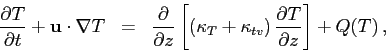where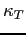is the molecular diffusivity (defined in suntans.dat) and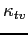is the vertical turbulent eddy-diffusivity, computed in turbulence.c. terms to simplify the discussion, the temperature equation is given by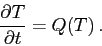(1)

The source term can be linearized about some temperature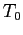so that, defining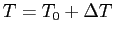, we have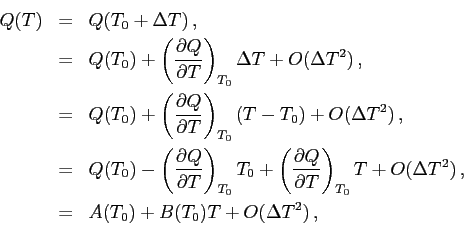where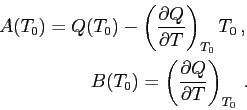The heat equation is discretized with the theta method so that equation (1) becomes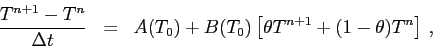where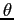is specified by the value of theta in suntans.dat. After rearranging, the temperature at the new time step is obtained with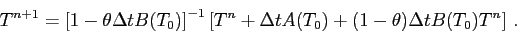Typically the best linearization is to linearize about the temperature at time step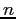so that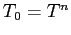. A simple implementation of heat flux is provided in the windstress example in suntans/main/examples/windstress and the code is in the HeatSource() function in suntans/main/examples/windstress/sources.c.Next: Restarting Runs Up: Specifying initial and boundary Previous: No-slip boundary conditions   Contents
2014-08-06